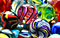# Marble Testing RxJS - throttleTime | debounceTime | bufferTime | buffer

Some examples to help you keep your marblesmaybe in the future we’ll be visualizing our marble tests in 3D

The other day I wanted to add test coverage for a custom-built Pipeable RxJS Operator used for some touch screen input events. This is what the operator looked like:

`const customBuffer = <T>(time: number): OperatorFunction<T, T[]> => ((source: Observable<T>): Observable<T[]> => source.pipe(    buffer(source.pipe(      throttleTime(time),      debounceTime(time))    )));`

I decided to give jasmine-marbles a whirl.

It didn’t take long before I realized 2 things:

1. I didn’t understand what the error messages were trying to tell me.
2. The documentation wasn’t helping either.

Here’s an example of such an error message:

`Expected \$.length = 3 to equal 7. Expected \$.notification.value = [ 'a', 'b', 'c' ] to equal undefined. Expected \$.frame = 5 to equal 2. Expected \$.notification.value = [ 'd', 'e', 'f' ] to equal undefined. Expected \$.frame = 9 to equal 2. Expected \$.notification.kind = 'C' to equal 'N'. Expected \$.notification.hasValue = false to equal true. Expected \$ = undefined to equal Object({ frame: 9, notification: Notification({ kind: 'N', value: undefined, error: undefined, hasValue: true }) }). Expected \$ = undefined to equal Object({ frame: 9, notification: Notification({ kind: 'N', value: undefined, error: undefined, hasValue: true }) }). Expected \$ = undefined to equal Object({ frame: 9, notification: Notification({ kind: 'N', value: undefined, error: undefined, hasValue: true }) }). Expected \$ = undefined to equal Object({ frame: 17, notification: Notification({ kind: 'C', value: undefined, error: undefined, hasValue: false }) }). Error: Expected \$.length = 3 to equal 7. Expected \$.notification.value = [ 'a', 'b', 'c' ] to equal undefined. Expected \$.frame = 5 to equal 2. Expected \$.notification.value = [ 'd', 'e', 'f' ] to equal undefined. Expected \$.frame = 9 to equal 2. Expected \$.notification.kind = 'C' to equal 'N'. Expected \$.notification.hasValue = false to equal true. Expected \$ = undefined to equal Object({ frame: 9, notification: Notification({ kind: 'N', value: undefined, error: undefined, hasValue: true }) }). Expected \$ = undefined to equal Object({ frame: 9, notification: Notification({ kind: 'N', value: undefined, error: undefined, hasValue: true }) }). Expected \$ = undefined to equal Object({ frame: 9, notification: Notification({ kind: 'N', value: undefined, error: undefined, hasValue: true }) }). Expected \$ = undefined to equal Object({ frame: 17, notification: Notification({ kind: 'C', value: undefined, error: undefined, hasValue: false }) }).     at <Jasmine>`

Not able to make much sense of the error messages, I decided to take my operator apart and start by writing simple unit tests for the individual building blocks. With a some trial and error, here’s what I got.

`const getTestScheduler = () => new TestScheduler((actual, expected) => expect(actual).toEqual(expected));describe('marbles testing', () => {  it('should delay', () => {    getTestScheduler().run(({ cold, expectObservable }) => {      const time = 2;      const operation = delay(time);      const actual   = 'abcdef--|';      const expected = '--abcdef--|';      expectObservable(cold(actual).pipe(operation)).toBe(expected);    });  });  it('should throttleTime', () => {    getTestScheduler().run(({ cold, expectObservable }) => {      const time = 2;      const operation = throttleTime(time);      const actual   = 'abcdef---|';      const expected = 'a--d-----|';      expectObservable(cold(actual).pipe(operation)).toBe(expected);    });  });  it('should debounceTime', () => {    getTestScheduler().run(({ cold, expectObservable }) => {      const time = 2;      const operation = debounceTime(time);      const actual   = 'abcdef--|';      const expected = '-------f|';      expectObservable(cold(actual).pipe(operation)).toBe(expected);    });  });  it('should throttleTime and debounceTime', () => {    getTestScheduler().run(({ cold, expectObservable }) => {      const time = 2;      const operation1 = throttleTime(time);      const operation2 = debounceTime(time);      const actual   = 'abcdef---|';      const expected = '--a--d---|';      expectObservable(cold(actual).pipe(operation1, operation2)).toBe(expected);    });  });  it('should bufferTime', () => {    getTestScheduler().run(({ cold, expectObservable }) => {      const time = 2;      const operation = bufferTime(time);      const actual   = 'abcdef---|';      const expected = '--x-y-z-w(w|)';      const values = {        x: ['a', 'b'],        y: ['c', 'd', 'e'],        z: ['f'],        w: [],      };      expectObservable(cold(actual).pipe(operation)).toBe(expected, values);    });  });  it('should bufferTime without events', () => {    getTestScheduler().run(({ cold, expectObservable }) => {      const time = 2;      const operation = bufferTime(time);      const actual   = '---------|';      const expected = '--w-w-w-w(w|)';      const values = {        w: [],      };      expectObservable(cold(actual).pipe(operation)).toBe(expected, values);    });  });  it('should buffer', () => {    getTestScheduler().run(({ cold, expectObservable }) => {      const time = 2;      const operation = buffer(of(1).pipe(delay(time)));      const actual   = 'abcdef---|';      const expected = '--(x|)';      const values = {        x: ['a', 'b'],      };      expectObservable(cold(actual).pipe(operation)).toBe(expected, values);    });  });  it('should buffer with throttleTime and debounceTime', () => {    getTestScheduler().run(({ cold, expectObservable }) => {      const time = 2;      const actual   = 'abcdef---|';      const expected = '--x--y---|';      const values = {        x: ['a', 'b', 'c'],        y: ['d', 'e', 'f'],      };      const o\$ = cold(actual);      expectObservable(o\$.pipe(buffer(o\$.pipe(throttleTime(time), debounceTime(time))))).toBe(expected, values);    });  });  it('should not buffer with throttleTime and debounceTime', () => {    getTestScheduler().run(({ cold, expectObservable }) => {      const time = 2;      const actual   = '-------|';      const expected = '-------|';      const o\$ = cold(actual);      expectObservable(o\$.pipe(buffer(o\$.pipe(throttleTime(time), debounceTime(time))))).toBe(expected);    });  });});`

With these tests in place, I was then able to successfully write a test that covered my :

`describe('customBuffer', () => {  it('should emit in groups', () => {    getTestScheduler().run(({ cold, expectObservable }) => {      const time = 2;      const actual   = 'abcdef-----|';      const expected = '--x--y-----|';      const values = {        x: ['a', 'b', 'c'],        y: ['d', 'e', 'f'],      };      expectObservable(cold(actual).pipe(customBuffer(time))).toBe(expected, values);    });  });  it('should not emit in groups', () => {    getTestScheduler().run(({ cold, expectObservable }) => {      const time = 2;      const actual   = '-------|';      const expected = '-------|';      expectObservable(cold(actual).pipe(customBuffer(time))).toBe(expected);    });  });});`

And that’s it !

Ok, one last thing that might be useful to share. An error message like this:

`Expected \$.notification.kind = 'N' to equal 'C'`

means you expected the stream to close (with a ) when the actual stream was still emitting events. stands for , stands for and stands for .

If you have any questions, thoughts or suggestions, please leave them in the comments below.

And if you liked this article, please give it a 👏 !

I write about spirituality and personal development.

## More from Chris Dickinson

I write about spirituality and personal development.